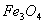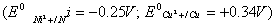Thursday 21st January 2021

CBSE Guess > Papers > Question Papers > Class XII > 2004 > Chemistry > Compartment Outside Delhi Set-II

CHEMISTRY (Set II—Compartmet Outside Delhi)

Except for the following questions, all the remaining questions have been asked in Set I.

Q. 1. What happens when ferrimagneticis heated at 850 K and why? 1

Q. 2. A and B liquids on mixing produce a warm solution. Which type of deviation from Raoult's law is there? 1

Q. 3. How is acetone obtained from 2-bromopropane? 1

Q. 4. How is phenol obtained from aniline? 1

Q. 6. Justify the following statements: 2
(a) An ezothermic reaction is always thermodynamically spontaneous.
(b) The entropy of a substance increases on going from liquid to vapour state at any temperature.

Q. 8. (a) The standard reduction potential for the Zn2+(aq) I Zn (s) half cell is 0.76 V. Write the reactions occu ring at the electrodes when coupled with standard hydro gen electrode (SHE).
(b) Can a nickel spatula be used to stir a solution of CuSO4 ? Support your answer with reason.2

Q. 10. Give reasons:
(a) Xenon does not form fluorides such as XeF3 and XeF5 .
(b) Out of noble gases, only Xenon is known to form real chemical compounds.

Q. 13. Justify the following statements: 3

Q. 23. (a) List two major classes of antibiotics and give one example of each class.
(b) Write one example of each of the following:
(i) Azo dye (ii) Anthraquinone dye 3

Q. 25. Name the chief ores of zinc and mercury. Describe the reactions involved in the extraction of these metals from the respective ores. 5

 Chemistry 2004 Question Papers Class XII Delhi Outside Delhi Compartment Delhi Compartment Outside DelhiSet ISet ISet ISet ISet IISet IISet IISet IISet IIISet III

CBSE 2004 Question Papers Class XII